• 卷积神经网络如何进行图像识别
千次阅读
2020-04-27 11:03:21

在机器视觉的概念中，图像识别是指软件具有分辨图片中的人物、位置、物体、动作以及笔迹的能力。计算机可以应用机器视觉技巧，结合人工智能以及摄像机来进行图像识别。

 什么是图像识别?为什么要进行图像识别?     在机器视觉的概念中，图像识别是指软件具有分辨图片中的人物、位置、物体、动作以及笔迹的能力。计算机可以应用机器视觉技巧，结合人工智能以及摄像机来进行图像识别。     对于人类和动物的大脑来说，识别物体是很简单的，但是同样的任务对计算机来说却是很难完成的。当我们看到一个东西像树、或者汽车、或者我们的朋友，我们在分辨他是什么之前，通常不需要下意识的去研究他。然而，对于计算机来说，辨别任何事物(可能是钟表、椅子、人或者动物)都是非常难的问题，并且找到问题解决方法的代价很高。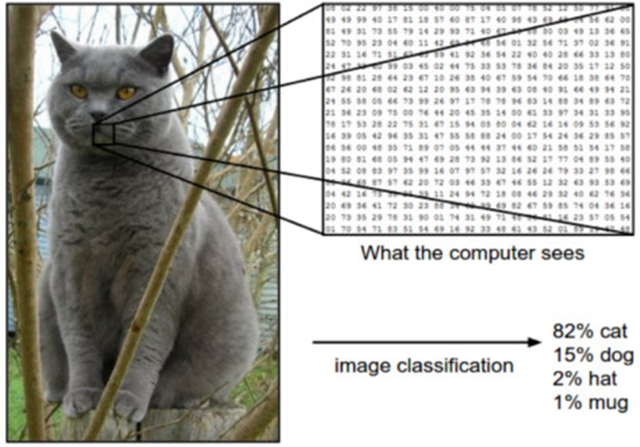图像识别算法一般采用机器学习方法，模拟人脑进行识别的方式。根据这种方法，我们可以教会计算机分辨图像中的视觉元素。计算机依靠大型数据库，通过对数据呈现的模式进行识别，可以对图像进行理解，然后形成相关的标签和类别。     图像识别技术的普及应用     图像识别技术有许多应用。其中最常见的就是图像识别技术助力的人物照片分类。谁不想更好地根据视觉主题来管理巨大的照片库呢?小到特定的物品，大到广泛的风景。     图片识别技术赋予了照片分类应用的用户体验新感受。除了提供照片存储，应用程序也可以更进一步，为人们提供更好的发现和搜索功能。有了通过机器学习进行自动图像管理的功能，它们就可以做到这一点。在应用程序中整合的图像识别程序界面可以根据机器所鉴定的特征对图像进行分类，并且根据主题将照片分组。     图像识别的其他应用包括存储照片和视频网站、互动营销以及创意活动，社交网络的人脸和图像识别，以及具有大型视觉图像库网站的图像分类。     图像识别是一项艰巨的任务     图像识别不是一项容易的任务，一个好的方法是将元数据应用到非结构数据上。聘请专家对音乐和电影库进行人工标注或许是一个令人生畏的艰巨任务，然而有的挑战几乎是不可能完成的，诸如教会无人驾驶汽车的导航系统将过马路的行人与各种各样的机动车分辨出来，或者将用户每天传到社交媒体上的数以百万计的视频或照片进行标注以及分类。     解决这个问题的一个方法是使用神经网络。理论上，我们可以使用传统神经网络对图像进行分析，但是实际上从计算角度来看代价很高。举个例子，一个传统的神经网络在处理一张很小的图片时(假设30*30像素)仍然需要50万个参数以及900个输入神经元。一个相当强大的机器可以运行这个网络，但是一旦图片变大了(例如500*500像素)，参数以及输入的数目就会达到非常高的数量级。     神经网络应用于图像识别的另一个会出现的问题是：过拟合。简单地说，过拟合一般发生在模型过于贴合训练数据的情况下。一般而言，这会导致参数增加(进一步增加了计算成本)以及模型对于新数据的结果在总体表现中有所下降。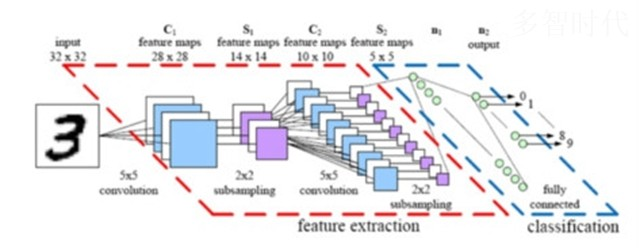卷积神经网络结构模型     根据神经网络的构建方式，一个相对简单的改变就可以让较大的图像变得更好处理。改变的结果就是我们所见到的卷积神经网络(CNNs，ConvNets)。     神经网络的广适性是他们的优点之一，但是在处理图像时，这个优点就变成了负担。卷积神经网络对此专门进行了折衷：如果一个网络专为处理图像而设计，有些广适性需要为更可行的解决方案做出让步。     对于任意图像，像素之间的距离与其相似性有很强的关系，而卷积神经网络的设计正是利用了这一特点。这意味着，对于给定图像，两个距离较近的像素相比于距离较远的像素更为相似。然而，在普通的神经网络中，每个像素都和一个神经元相连。在这种情况下，附加的计算负荷使得网络不够精确。     卷积神经网络通过消除大量类似的不重要的连接解决了这个问题。技术上来讲，卷积神经网络通过对神经元之间的连接根据相似性进行过滤，使图像处理在计算层面可控。对于给定层，卷积神经网络不是把每个输入与每个神经元相连，而是专门限制了连接，这样任意神经元只能接受来自前一层的一小部分的输入(例如3*3或5*5)。因此，每个神经元只需要负责处理一张图像的一个特定部分。(顺便提一下，这基本就是人脑的独立皮质神经元工作的方式。每个神经元只对完整视野的一小部分进行响应)。     卷积神经网络的工作过程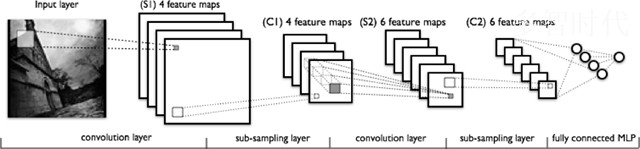上图从左到右可以看出： 网络对输入的真实图像进行扫描提取特征。传递特征的滤波器由浅色方块表示。 激活图由堆栈形式排列，每一个对应所用的滤波器。较大的方形是要进行下采样的块。 激活图通过下采样进行压缩。 下采样后的激活图经过滤波器产生新的一组激活图。 第二次下采样——对第二组激活图进行压缩。 全连接层为每个节点的输出指定一个标签。      卷积神经网络的滤波器如何对连接根据相似性进行滤波?诀窍在于新加的两种层结构：池化层和卷积层。我们下面将步骤进行分解。用为了只完成一件事情而设计的网络实例进行介绍，即决定一张图片中是否含有一个老爷爷。   过程的第一步是卷积层，它自己本身就包含几个小步骤。 首先，我们要将包含老爷爷的图片分解为一系列有重叠的3*3的像素块。 在这之后，我们将每个像素块输入一个简单的、单层的神经网路，保持权重不变。这一步将像素块集转化成一个矩阵。只要我们保持每块像素块都比较小(这里是3*3)，处理它们所需的网络也可以保持可控以及小型。 下一步，输出值会被排成矩阵，以数据形式表示照片中每个区域的内容，不同轴分别代表颜色、宽度、和高度通道。对于每一个图像块，都有一个3*3*3的表示。(如果要处理视频，可以加入第四维度代表时间)。       接下来是池化层。池化层对这些3或4维的矩阵在空间维度上进行下采样。处理结果是池化阵列，其中只包含重要部分图像，并且丢弃了其他部分，这样一来最小化了计算成本，同时也能避免过拟合问题。    经过下采样的矩阵作为全连接层的输入。由于经过了池化和卷积操作，输入的尺寸被大幅减小，我们现在有了正常网络能处理的，同时能保持数据最重要特性的东西。最后一步的输出代表系统对于图片中有老爷爷这一判断的确信度。     在实际应用中，卷积神经网络的工作过程很复杂，包括大量的隐藏、池化和卷积层。除此之外，真实的卷积神经网络一般会涉及上百甚至上千个标签，而不只是样例中的一个。     如何搭建卷积神经网络 从头开始构建一个卷积神经网络是很费时费力的工作。目前已经有了许多API能够实现关于卷积神经网络的想法，而不需要工程师去了解机器学习的原理或者计算机视觉的专业知识。 Google云视觉     Google云视觉是使用REST  API搭建的视觉识别API。它基于开源的TensorFlow框架。它可以检测到独立的人脸或物体，并且包含十分全面的标签集。 IBM Watson 视觉识别     IBM Watson 视觉识别是Waston Developer  Cloud服务的一部分，并且自带大量内置类别，但它实际是为训练基于你提供图片的自定义类别而打造的。同时，和Google云视觉一样，它也提供了大量花哨的特性，包括NSFW以及OCR检测。 Clarif.ai     Clarif.ai也是一个使用REST  API的初创图像识别服务。关于Clarif.ai有趣的一点是，它自带的一系列模块可以用于修改算法，将其应用到特定的主题上，例如食物、旅游和结婚。     尽管上述的API适合一些一般的应用，但最好还是针对特定问题开发一个自定义的解决方案。幸运的是，大量可用的库解决了优化和计算方面的问题，开发人员和数据科学家可以只关注训练模型，这样一来他们的工作便轻松了一些。这些库包括Theano、  Torch、 DeepLearning4J以及TensorFlow，已成功地运用在各种各样的应用程序中。 卷积神经网络的有趣小应用：自动为无声电影添加声音      要为无声电影添加匹配的声音，系统必须在这个任务中自动合成声音。该系统使用上千个视频样例进行训练，视频带有鼓棍敲打不同表面产生的不同声音。一个深度学习模型将视频的帧和预录的声音建立联系，然后选择能够完美匹配场景的音频进行播放。系统会通过图灵测试进行评估，让人来决定那个视频是合成的，哪个是真实的声音。这是卷积神经网络和LSTM循环神经网络的一个很潮的应用。 在不久的将来，多智时代一定会彻底走入我们的生活，有兴趣入行未来前沿产业的朋友，可以收藏多智时代，及时获取人工智能、大数据、云计算和物联网的前沿资讯和基础知识，让我们一起携手，引领人工智能的未来！
更多相关内容
• 卷积神经网络图像识别python代码pdf；卷积神经网络图像识别python代码pdfpython
• 基于卷积神经网络图像识别算法，基于卷积神经网络图像识别算法PPT,基于卷积神经网络图像识别算法课件
• 基于卷积神经网络图像识别的港口防火系统设计.pdf
• 基于卷积神经网络图像识别 基于卷积神经网络图像识别
• 基于深度学习卷积神经网络图像识别技术的研究与应用.pdf
• 这是一篇关于深度学习应用于图像处理的高质量文献，其中的方法比较新颖。
• 基于非线性修正函数的卷积神经网络图像识别研究.pdf
• 基于LeNet-5改进的卷积神经网络图像识别方法.pdf
• 不同数据集容量下的卷积神经网络图像识别性能.pdf
• 深度卷积神经网络图像识别模型对抗鲁棒性技术综述.pdf
• 基于矩阵2-范数池化的卷积神经网络图像识别算法.pdf
• 卷积神经网络图像识别技术在入侵监测系统中的应用研究.pdf
• 基于深度学习卷积神经网络图像识别技术的研究分析探讨.pdf
• 基于卷积神经网络图像识别，网络结构为lenet5，在训练集上的正确率可以达到90+，测试集正确率在60-70%。
• 基于卷积神经网络图像识别的智能电子秤系统.pdf
• 基于卷积神经网络的食物图像识别，提供数据集下载，使用python、TensorFlow等等。
• 卷积神经网络特别适合处理像图片、视频、音频、语言文字等，这些与相互位置有一定关系的数据。 卷积神经网络（Convolutional Nerual Network,CNN） 为什么计算机可以处理图片--因为在计算机语言中图片可以用数字...

# 目录

卷积神经网络（Convolutional Nerual Network,CNN）

卷积层定义

卷积层计算的代码实现

# 卷积神经网络（Convolutional Nerual Network,CNN）

卷积神经网络特别适合处理像图片、视频、音频、语言文字等，这些与相互位置有一定关系的数据。

卷积神经网络与多连接神经网络一样，都属于神经网络的一种类别，只是多连接神经网络的隐藏层的特点是称为多连接层的该层上的每一个节点与上一层的全部节点是全连接的关系，卷积神经网络的隐藏层上的每一个节点与上一层的全部节点直接并非是全部连接的关系，而是仅与一部分的节点有连接。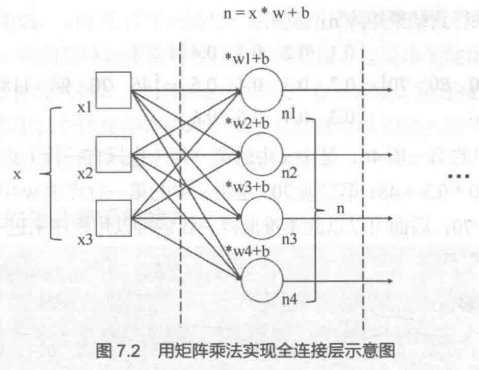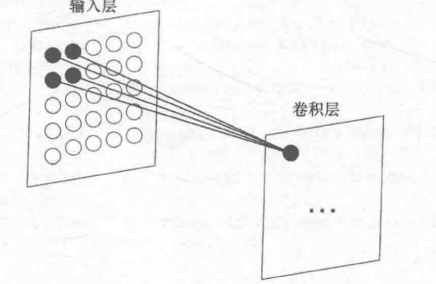# 为什么计算机可以处理图片

### --因为在计算机语言中图片可以用数字化，用四维数组来表示

既然卷积神经网络可以处理图片，那么我们就要理解图片在计算机语言中是如何表达的。图片其实是“点阵”图，由一个个点按照一定的顺序组合而成，那我们就可以联想到一个概念--数组。

图片可以分为三类：纯黑白图片、灰度图片、彩色图片

关于图片数字化，以最简单的纯黑白图片为例，我们可以用二维数组--矩阵来表示，比如h×w，高度h代表该点阵有多少行，也可以理解成每列有多少点；宽度w代表该点阵每行有多少点；通常我们说的摄像头是多少万像素，其实就是这个摄像头拍出来的照片保存成“点阵”图后的高度h和宽度w相乘的结果，如1900万×1250万这种写法。

上面说的是矩阵的维度，下面说矩阵中每个点的值所代表的含义。矩阵中每一个点一般叫“像素”，其值叫“像素值”，对于纯黑白图片，其像素值可以用0或1表示，0代表该点是白色，1代表该点是黑色；对于灰度图片，其像素值可以用[0,255]范围内的一个数字来代表黑色的深浅程度；对于彩色图片，每个点会用一个向量来表示，如RGB，即这个“点”此时是一个三维向量，RGB分别代表红色、绿色和蓝色，通常把这三项叫做该“点”颜色的通道，即分别为R通道、G通道、B通道。

这就意味着，世界上所有的图片，都可以数字化！图片数字化以后，就可以利用计算机语言中的数组来表达！这就是图片可以用计算机处理的第一步！

如下图，数字“2”的一个最简单的6×6的纯黑白图片的点阵图为：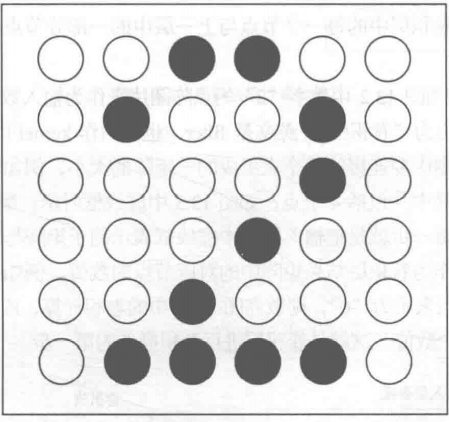该点阵图的数字化表达为（用二维数组进行表达）：

[[0,0,1,1,0,0],
[0,1,0,0,1,0],
[0,0,0,0,1,0],
[0,0,0,1,0,0],
[0,0,1,0,0,0],
[0,1,1,1,1,0]]

# 卷积层定义

同全连接层一样，卷积层也是神经网络中包含某一特点的一个隐藏层。该隐藏层的作用就是对输入层做卷积。要了解卷积层需要了解两个概念，一是卷积核，另一个是卷积运算。

卷积核是一个数字矩阵（英文是filter，或者kernel），卷积核的大小即该数字矩阵的维度。

卷积运算，见下图，卷积核与左侧的虚线框内的子矩阵先点乘，后求和。这个运算就是卷积运算。之后卷积核会继续与输入矩阵的第二个子矩阵进行同样的卷积运算。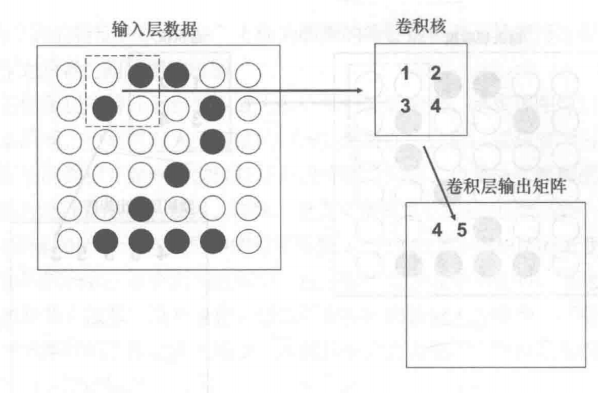得到的卷积层输出矩阵有两种情况，①不填充节点0，则得到的输出矩阵维数会减小；②填充节点0，则得到的输出矩阵维数不变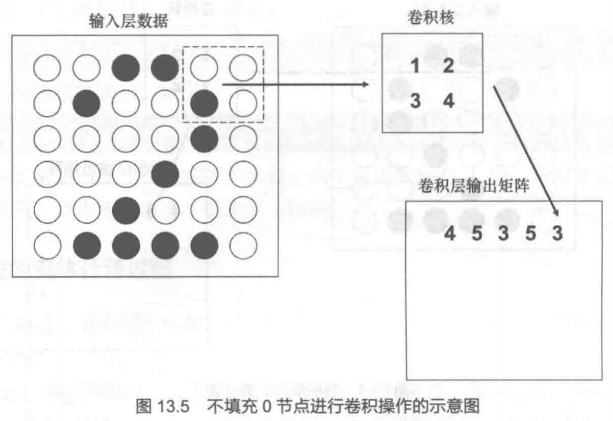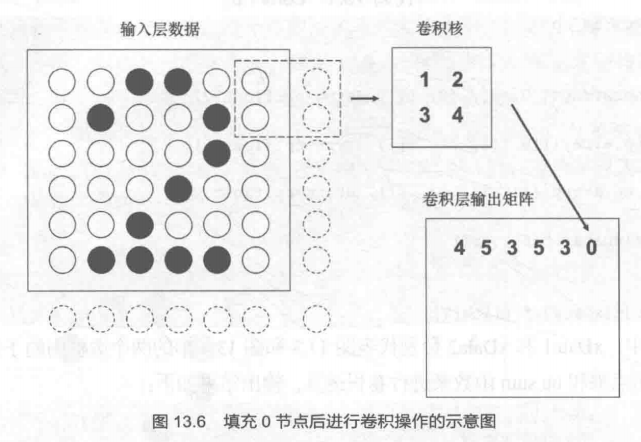理解一下卷积运算的好处

①降低数据维度，不填充节点0，得到的输出矩阵维数会减小

②特征提取：卷积运算相当于把原图中相邻几个节点一起进行运算，得到一个数字，该数字包含了这几个节点的【综合数值】+【相对位置的信息】的特征（这是一个特征提取的过程）。举个栗子，图13.5中的数字5，在一定程度上代表了“2”字“向右上方转折”这一笔画特征，这是输入层的单个点所不能包含的信息。

③降低计算复杂度，利用上面的特征提取，可以利用特征来对图像进行识别，能比单点识别的运算量小。举个栗子，图13.5中的数字5，代表“2”字“向右上方转折”这一笔画特征，如果某图片的数字是“1”，进行卷积运算后得到的输出层相同位置的数字不是5，即可判断出，该数字不是2。而不用长篇幅的单点识别对比。通过特征提取，这个特征能用来识别是不是数字“2”的重要依据之一。

④增加卷积层的数量，可以把低级别的特征逐步提取成为高级别特征。----这是卷积层的最重要的能力！！

# 卷积层计算的代码实现

import tensorflow as tf
xData=tf.constant([[,,,,,],[,,,,,],
[,,,,,],[,,,,,],
[,,,,,],[,,,,,]],dtype=tf.float32)
kernel=tf.constant([[,],[,]],dtype=tf.float32)
sess=tf.Session()
result=sess.run(y)
print(y)

解释一下代码所用到的语句的意思

tf.constant是tf中生成一个不会改变的张量的函数，即常量张量

tf.nn.conv2d的四个命名参数：
input是输入数据，input要求输入的数据是一个四维数组，上面xData我们定义的是一个三维数组，通过tf.reshape把三维的数组变成四维的数组输入给input。
其中第一个维度代表一次要处理的图片数量，第二、三个维度代表输入图片的h和w，第四个维度代表图片彩色的通道数。
这里我们把xData reshape成[1,6,6,1]，即一次处理一张图片，该图片的h和w分别为6、6，黑白图片的通道数为1，把xData reshape后满足参数input的要求。
即记住input实际输入的是一个四维数组
简记input=[一次处理图片数量，h，w，通道数量]

filter是卷积核，要求输入的数据也是一个四维数组，简记filter=[h,w,输入通道数，输出通道数]
其中输入通道数和输出通道数一般都是相等的。
对于上面代码filter=tf.reshape(kernel,[2,2,1,1])中[2,2,1,1]的理解可以参照上段对input的理解。
且记住filter实际输入的是一个四维数组

strides=[,,,]输入的是一个四维的向量，用于指定卷积时卷积核移动的步长。
每个维度分别对应在输入数组(input)4个维度上的步长。
举个栗子：striders=[1,2,3,1]，即代表对输入数组(input)，每次处理1张图片，每张图片隔2步横着走，隔3步竖着走，在单个通道内走1步

padding只有两个值：'VALID' or 'SAME',前者代表不填充节点0，后者代表填充节点0

多说一句~这里提供的代码时1.x版本的tensorflow可运行的代码，如果安装的2.x版本的tensorflow可以在上段代码的前面加上下面两句话（本意是导入1.x版本的tensorflow且使得2.x版本的语句失效，不过这个方法会出现警告，有一天可能会失效~~）

import tensorflow.compat.v1 as tf
tf.disable_v2_behavior()

disable_resource_variables (from tensorflow.python.ops.variable_scope) is deprecated and will be removed in a future version.
Instructions for updating:
non-resource variables are not supported in the long term

#即disable_resource_variables在未来的版本中会被去掉

以上为作者学习《深度学习--基于Python语言和TensorFlow平台(视频讲解版)》对卷积神经网络的复习、理解加总结，如需学习更详尽的知识，请参考本书。

展开全文python tensorflow
• CNN卷积神经网络图像识别 前言 神经网络(neual networks)是人工智能研究领域的一部分，当前最流行的神经网络是深度卷积神经网络(deep convolutional neural networks, CNNs)，虽然卷积网络也存在浅层结构，但是...

# CNN卷积神经网络及图像识别

## 前言

神经网络(neual networks)是人工智能研究领域的一部分，当前最流行的神经网络是深度卷积神经网络(deep convolutional neural networks, CNNs)，虽然卷积网络也存在浅层结构，但是因为准确度和表现力等原因很少使用。目前提到CNNs和卷积神经网络，学术界和工业界不再进行特意区分，一般都指深层结构的卷积神经网络，层数从”几层“到”几十上百“不定。

CNNs目前在很多很多研究领域取得了巨大的成功，例如: 语音识别，图像识别，图像分割，自然语言处理等。虽然这些领域中解决的问题并不相同，但是这些应用方法都可以被归纳为:

CNNs可以自动从(通常是大规模)数据中学习特征，并把结果向同类型未知数据泛化。

## 卷积神经网络-CNN 的基本原理

### 网络结构

基础的CNN卷积(convolution), 激活(activation), and 池化(pooling)三种结构组成。CNN输出的结果是每幅图像的特定特征空间。当处理图像分类任务时，我们会把CNN输出的特征空间作为全连接层或全连接神经网络(fully connected neural network, FCN)的输入，用全连接层来完成从输入图像到标签集的映射，即分类。当然，整个过程最重要的工作就是如何通过训练数据迭代调整网络权重，也就是后向传播算法。目前主流的卷积神经网络(CNNs)，比如VGG, ResNet都是由简单的CNN调整，组合而来。

典型的 CNN 由3个部分构成：

1. 卷积层：卷积层负责提取图像中的局部特征；
2. 池化层：池化层用来大幅降低参数量级(降维)；
3. 全连接层：全连接层类似传统神经网络的部分，用来输出想要的结果。

### 卷积

在CNN中，卷积可以近似地看作一个特征提取算子，简单来说就是，提取图片纹理、边缘等特征信息的滤波器。下面，举个简单的例子，解释一下特征提取算子是怎么工作的：比如有一张猫图片，人类在理解这张图片的时候，可能观察到圆圆的眼睛，可爱的耳朵，于是，判断这是一只猫。但是，机器怎么处理这个问题呢？传统的计算机视觉方法，通常设计一些算子（特征提取滤波器），来找到比如眼睛的边界，耳朵的边界，等信息，然后综合这些特征，得出结论——这是一只猫。

卷积神经网络，做了类似的事情，所谓卷积，就是把一个算子在原图上不断滑动，得出滤波结果——这个结果，我们叫做“特征图”（Feature Map），这些算子被称为“卷积核”(Convolution Kernel)。不同的是，我们不必人工设计这些算子，而是使用随机初始化，来得到很多卷积核（算子），然后通过反向传播，优化这些卷积核，以期望得到更好的识别结果。

卷积的运算过程可以用下图来表示：上面的卷积过程，没有考虑彩色图片有rgb三维通道（Channel），如果考虑rgb通道，那么，每个通道，都需要一个卷积核：当输入有多个通道时，我们的卷积核也需要有同样数量的通道。以上图为例，输入有RGB三个通道，我们的就卷积核，也有三个通道，只不过计算的时候，卷积核的每个通道，在对应通道滑动（卷积核最前面的通道在输入图片的红色通道滑动，卷积核中间的通道在输入图片的绿色通道滑动，卷积核最后面的通道在输入图片的蓝色通道滑动），如果我们想将三个通道的信息合并，可以将三个通道的计算结果相加得到输出。注意，输出只有一个通道。

卷积层的作用：

1. 提取图像的特征，并且卷积核的权重是可以学习的，卷积操作能突破传统滤波器的限制，根据目标函数提取出想要的特征；
2. 参数共享，降低了网络参数，提升训练效率。

### 池化

池化（Pooling），有的地方也称汇聚，实际是一个下采样（Down-sample）过程。由于输入的图片尺寸可能比较大，这时候，我们需要下采样，减小图片尺寸。池化层可以减小模型规模，提高运算速度，同时提高所提取特征的鲁棒性。

本文主要介绍最大池化（Max Pooling）和平均池化（Average Pooling）。

所谓最大池化，就是选取一定区域中数值最大的那个保留下来。比如区域大小为2*2，步长为2的池化过程如下（左边是池化前，右边是池化后），对于每个池化区域都取最大值：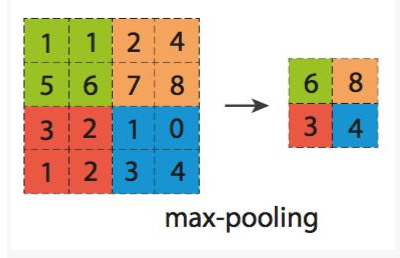最大池化最为常用，并且一般都取2*2的区域大小且步长为2。

平均池化则是取每个区域的均值，下图展示了两种池化的对比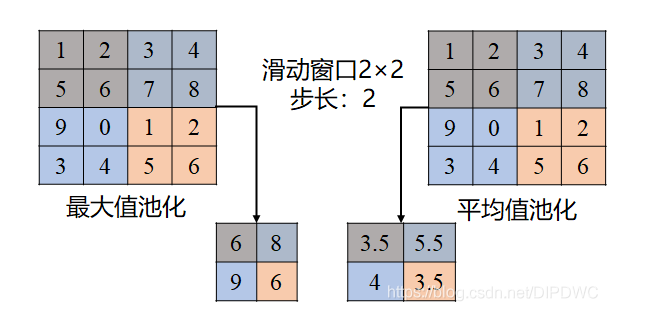### 全连接层

全连接层就是把卷积层和池化层的输出展开成一维形式，在后面接上与普通网络结构相同的回归网络或者分类网络，一般接在池化层后面，这一层的输出即为我们神经网络运行的结果。

## 代码示例

下面以AlexNet为例子，给出一个详细的卷积神经网络架构，首先AlexNet架构如下图所示：代码如下：

import tensorflow as tf
import numpy as np
# 定义各层功能
# 最大池化层
def maxPoolLayer(x, kHeight, kWidth, strideX, strideY, name, padding = "SAME"):
"""max-pooling"""
return tf.nn.max_pool(x, ksize = [1, kHeight, kWidth, 1],
strides = [1, strideX, strideY, 1], padding = padding, name = name)

# dropout
def dropout(x, keepPro, name = None):
"""dropout"""
return tf.nn.dropout(x, keepPro, name)

# 归一化层
def LRN(x, R, alpha, beta, name = None, bias = 1.0):
"""LRN"""
return tf.nn.local_response_normalization(x, depth_radius = R, alpha = alpha,
beta = beta, bias = bias, name = name)

# 全连接层
def fcLayer(x, inputD, outputD, reluFlag, name):
"""fully-connect"""
with tf.variable_scope(name) as scope:
w = tf.get_variable("w", shape = [inputD, outputD], dtype = "float")
b = tf.get_variable("b", [outputD], dtype = "float")
out = tf.nn.xw_plus_b(x, w, b, name = scope.name)
if reluFlag:
return tf.nn.relu(out)
else:
return out

# 卷积层
def convLayer(x, kHeight, kWidth, strideX, strideY,
featureNum, name, padding = "SAME", groups = 1):
"""convolution"""
channel = int(x.get_shape()[-1])
conv = lambda a, b: tf.nn.conv2d(a, b, strides = [1, strideY, strideX, 1], padding = padding)
with tf.variable_scope(name) as scope:
w = tf.get_variable("w", shape = [kHeight, kWidth, channel/groups, featureNum])
b = tf.get_variable("b", shape = [featureNum])

xNew = tf.split(value = x, num_or_size_splits = groups, axis = 3)
wNew = tf.split(value = w, num_or_size_splits = groups, axis = 3)

featureMap = [conv(t1, t2) for t1, t2 in zip(xNew, wNew)]
mergeFeatureMap = tf.concat(axis = 3, values = featureMap)
# print mergeFeatureMap.shape
return tf.nn.relu(tf.reshape(out, mergeFeatureMap.get_shape().as_list()), name = scope.name)

class alexNet(object):
"""alexNet model"""
def __init__(self, x, keepPro, classNum, skip, modelPath = "bvlc_alexnet.npy"):
self.X = x
self.KEEPPRO = keepPro
self.CLASSNUM = classNum
self.SKIP = skip
self.MODELPATH = modelPath
#build CNN
self.buildCNN()

# 构建AlexNet
def buildCNN(self):
"""build model"""
conv1 = convLayer(self.X, 11, 11, 4, 4, 96, "conv1", "VALID")
lrn1 = LRN(conv1, 2, 2e-05, 0.75, "norm1")
pool1 = maxPoolLayer(lrn1, 3, 3, 2, 2, "pool1", "VALID")

conv2 = convLayer(pool1, 5, 5, 1, 1, 256, "conv2", groups = 2)
lrn2 = LRN(conv2, 2, 2e-05, 0.75, "lrn2")
pool2 = maxPoolLayer(lrn2, 3, 3, 2, 2, "pool2", "VALID")

conv3 = convLayer(pool2, 3, 3, 1, 1, 384, "conv3")

conv4 = convLayer(conv3, 3, 3, 1, 1, 384, "conv4", groups = 2)

conv5 = convLayer(conv4, 3, 3, 1, 1, 256, "conv5", groups = 2)
pool5 = maxPoolLayer(conv5, 3, 3, 2, 2, "pool5", "VALID")

fcIn = tf.reshape(pool5, [-1, 256 * 6 * 6])
fc1 = fcLayer(fcIn, 256 * 6 * 6, 4096, True, "fc6")
dropout1 = dropout(fc1, self.KEEPPRO)

fc2 = fcLayer(dropout1, 4096, 4096, True, "fc7")
dropout2 = dropout(fc2, self.KEEPPRO)

self.fc3 = fcLayer(dropout2, 4096, self.CLASSNUM, True, "fc8")

wDict = np.load(self.MODELPATH, encoding = "bytes").item()
#for layers in model
for name in wDict:
if name not in self.SKIP:
with tf.variable_scope(name, reuse = True):
for p in wDict[name]:
if len(p.shape) == 1:
#bias
sess.run(tf.get_variable('b', trainable = False).assign(p))
else:
#weights
sess.run(tf.get_variable('w', trainable = False).assign(p))

展开全文机器学习
• 知识预备： 需要了解卷积神经网络的基本原理与结构，熟悉pytorch的使用，csdn有很多介绍卷积神经网络的文章，可查阅。 算法设计思路： （1） 收集数据集，利用 python 的 requests 库和 bs4 进行网络爬虫，下载数据...

## 项目简介

目的： 实现昆虫的图像分类，同时该模型也可以用于其他图像的分类识别，只需传入相应的训练集进行训练，保存为另一个模型即可，进行调用使用。
配置环境： pycharm（python3.7），导入pytotch库
知识预备： 需要了解卷积神经网络的基本原理与结构，熟悉pytorch的使用，csdn有很多介绍卷积神经网络的文章，可查阅。
例如：

https://blog.csdn.net/yunpiao123456/article/details/52437794
https://blog.csdn.net/weipf8/article/details/103917202

算法设计思路：
（1） 收集数据集，利用 python 的 requests 库和 bs4 进行网络爬虫，下载数据集
（2） 搭建卷积神经网络
（3）对卷积神经网络进行训练
（4） 改进训练集与测试集，并扩大数据集
（5） 保存模型
（6） 调用模型进行测试

## 项目效果展示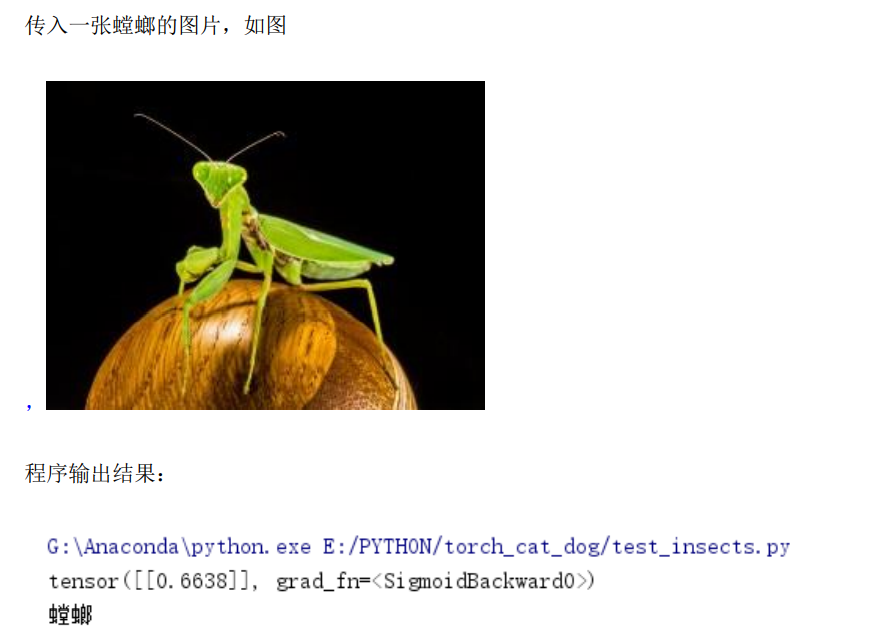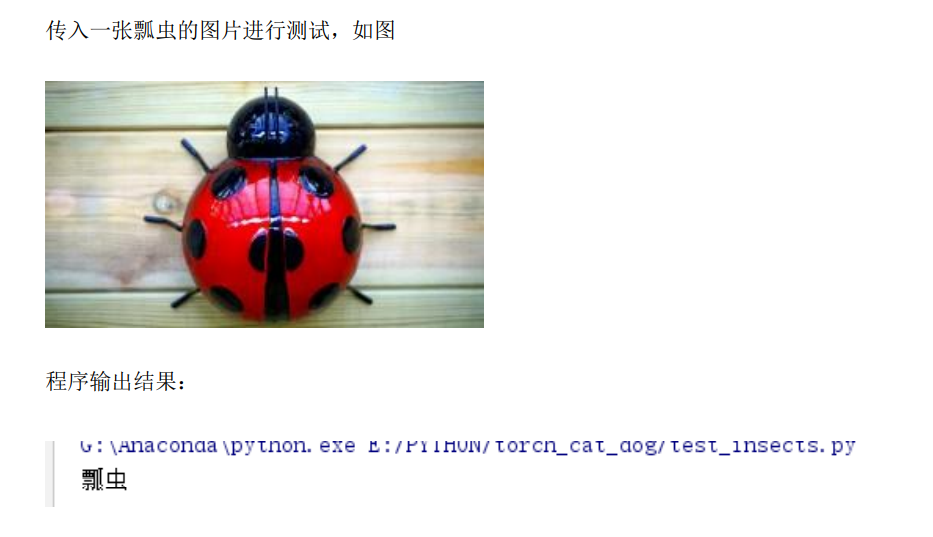注，模型我达到的最高正确率在85%，最后稳定在79%，中间出现了过拟合，可减少训练次数进行优化，数据集较少的情况下，建议训练10次就可。

## 程序运行流程图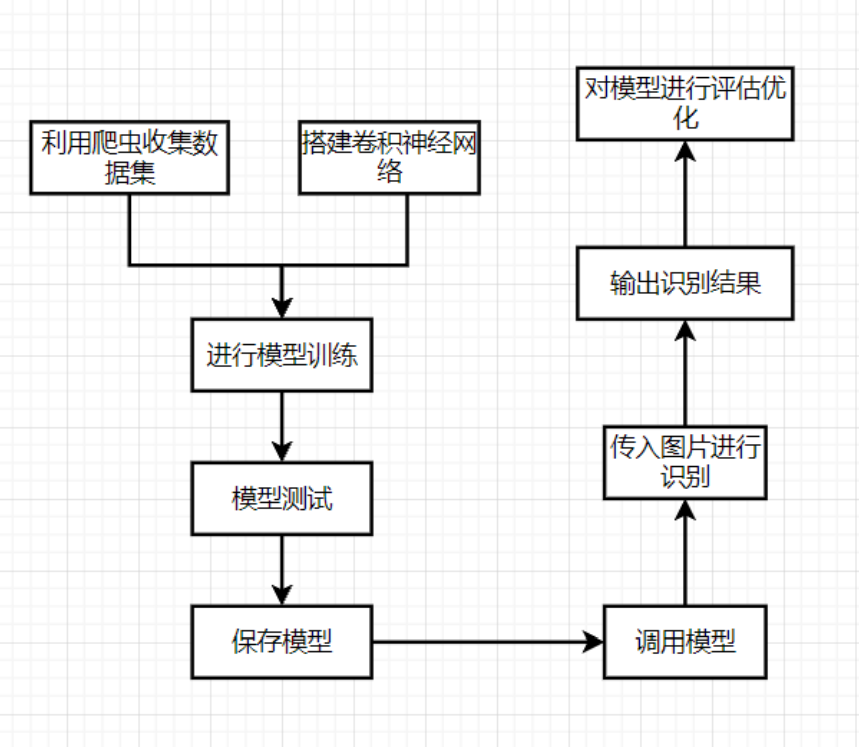## 代码使用说明

先训练模型，进行模型保存之后可对模型进行调用，不用每使用一次模型就要进行训练。文末有项目的完整代码：修改自己的数据集src位置，一般情况下能正常运行，如果不能，请检查自己的第三方库是否成功安装，以及是否成功导入。若有问题可以私信交流学习。

## 数据集准备

注：由于爬虫，会有一些干扰数据，所以我这里展示的是进行数据清洗之后的数据。
注：训练集：测试集=7：3（可自己修改）
注：若正确率不理想，可扩大数据集，数据清洗，图片处理等方面进行改进

### 训练集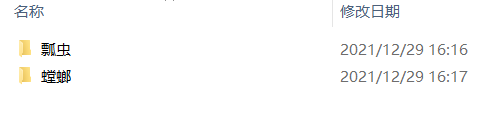部分数据展示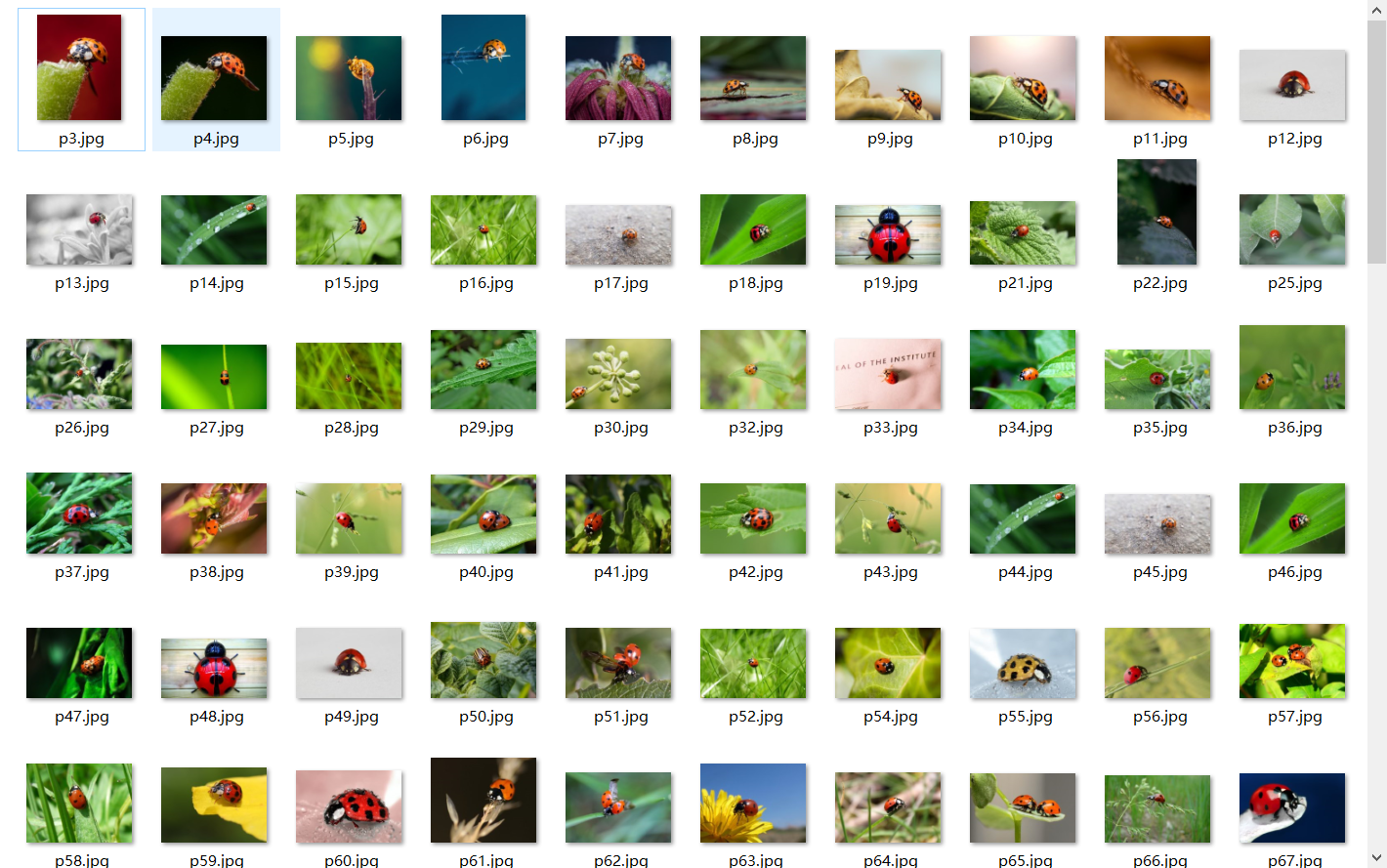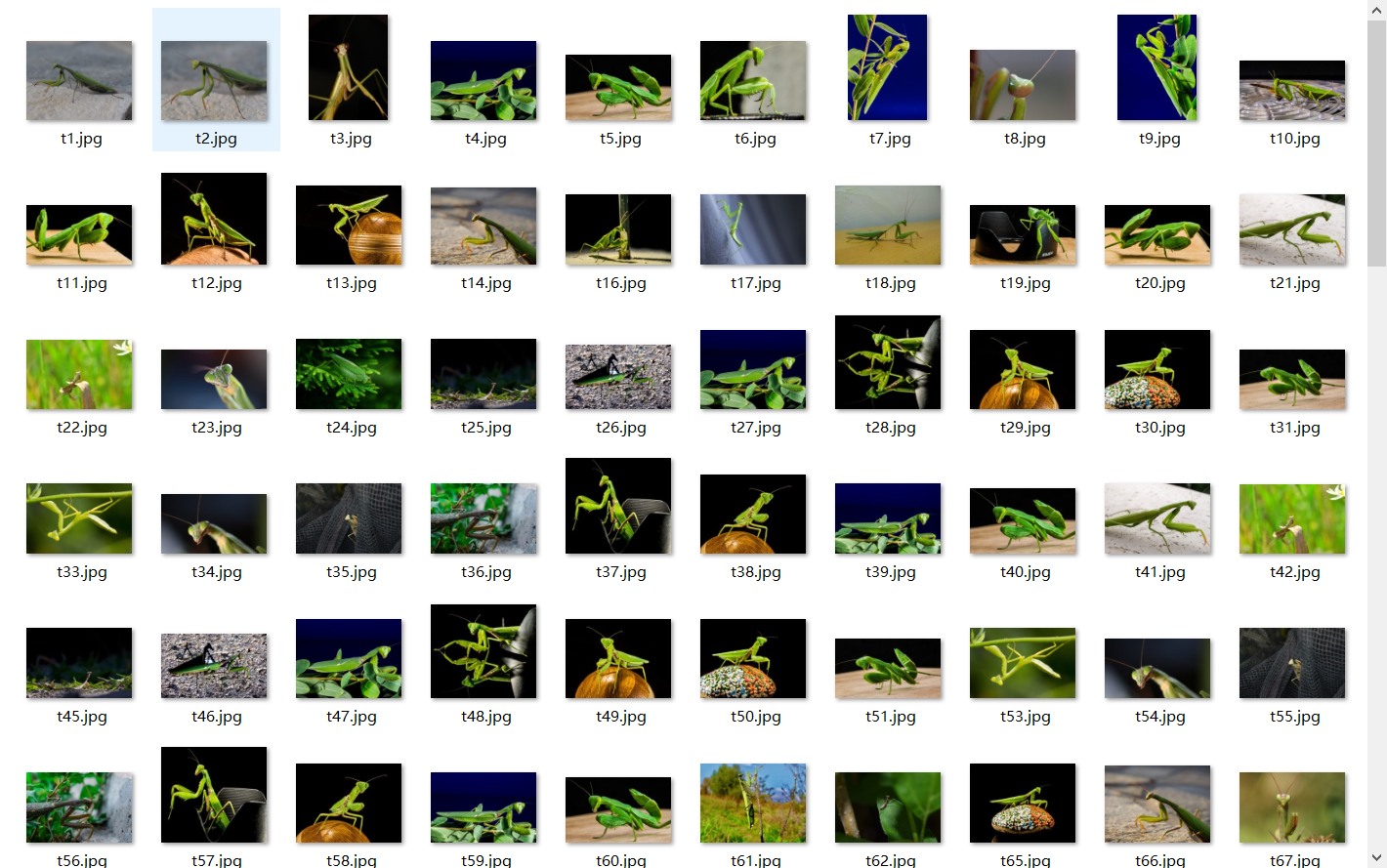文件格式与训练集一样。

## 搭建神经网络

框架：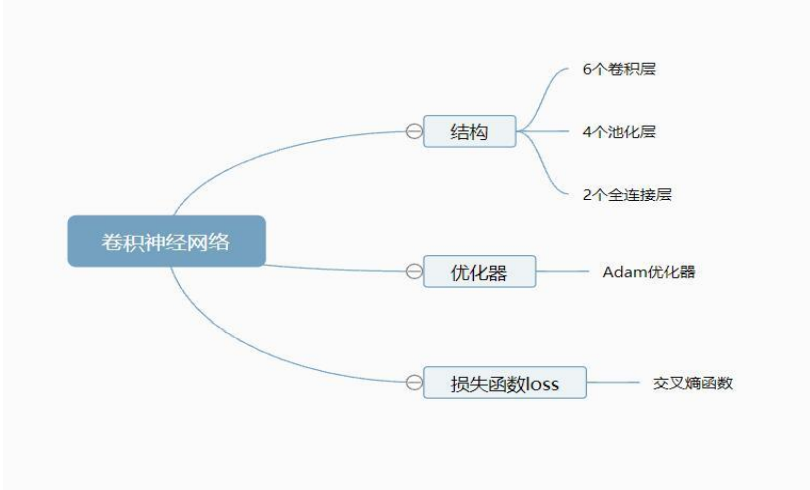结构：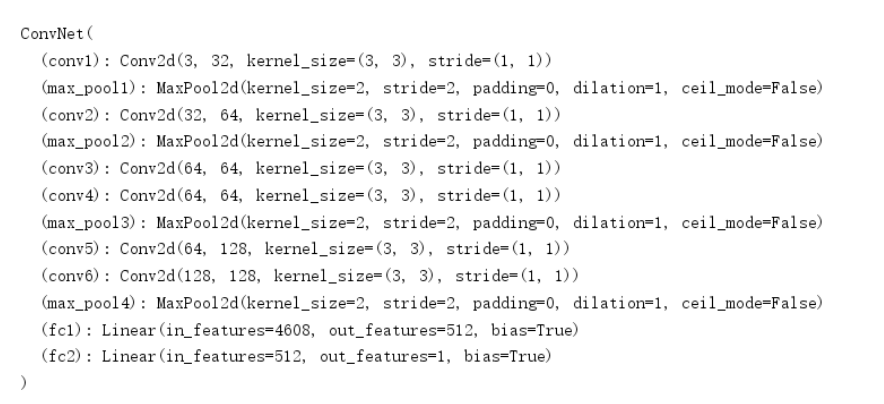代码实现：


# 定义网络
class ConvNet(nn.Module):
def __init__(self):
super(ConvNet, self).__init__()
self.conv1 = nn.Conv2d(3, 32, 3)
self.max_pool1 = nn.MaxPool2d(2)
self.conv2 = nn.Conv2d(32, 64, 3)
self.max_pool2 = nn.MaxPool2d(2)
self.conv3 = nn.Conv2d(64, 64, 3)
self.conv4 = nn.Conv2d(64, 64, 3)
self.max_pool3 = nn.MaxPool2d(2)
self.conv5 = nn.Conv2d(64, 128, 3)
self.conv6 = nn.Conv2d(128, 128, 3)
self.max_pool4 = nn.MaxPool2d(2)
self.fc1 = nn.Linear(4608, 512)
self.fc2 = nn.Linear(512, 1)

def forward(self, x):
in_size = x.size(0)
x = self.conv1(x)
x = F.relu(x)
x = self.max_pool1(x)
x = self.conv2(x)
x = F.relu(x)
x = self.max_pool2(x)
x = self.conv3(x)
x = F.relu(x)
x = self.conv4(x)
x = F.relu(x)
x = self.max_pool3(x)
x = self.conv5(x)
x = F.relu(x)
x = self.conv6(x)
x = F.relu(x)
x = self.max_pool4(x)
# 展开
x = x.view(in_size, -1)
x = self.fc1(x)
x = F.relu(x)
x = self.fc2(x)
x = torch.sigmoid(x)
return x


## 训练函数

def train(model, device, train_loader, optimizer, epoch):
model.train()
for batch_idx, (data, target) in enumerate(train_loader):

data, target = data.to(device), target.to(device).float().unsqueeze(1)

output = model(data)

# print(output)

loss = F.binary_cross_entropy(output, target)

loss.backward()

optimizer.step()

if (batch_idx + 1) % 1 == 0:
print('Train Epoch: {} [{}/{} ({:.0f}%)]\tLoss: {:.6f}'.format(

epoch, (batch_idx + 1) * len(data), len(train_loader.dataset),

100. * (batch_idx + 1) / len(train_loader), loss.item()))


## 测试函数

def test(model, device, test_loader):
model.eval()

test_loss = 0

correct = 0

data, target = data.to(device), target.to(device).float().unsqueeze(1)
# print(target)
output = model(data)
# print(output)
test_loss += F.binary_cross_entropy(output, target, reduction='mean').item()
pred = torch.tensor([ if num >= 0.5 else  for num in output]).to(device)
correct += pred.eq(target.long()).sum().item()

print('\nTest set: Average loss: {:.4f}, Accuracy: {}/{} ({:.0f}%)\n'.format(


## 模型-训练过程完整代码

### 模型保存使用的是torch.save(model，src),model即须保存的模型，src即模型保存的位置，后缀为pth

import torch.nn.functional as F
import torch.optim as optim
import torch
import torch.nn as nn
import torch.nn.parallel
from PIL import Image
import torch.optim
import torch.utils.data
import torch.utils.data.distributed
import torchvision.transforms as transforms
import torchvision.datasets as datasets

# 设置超参数
#每次的个数
BATCH_SIZE = 20
#迭代次数
EPOCHS = 10
#采用cpu还是gpu进行计算
device = torch.device('cuda' if torch.cuda.is_available() else 'cpu')

# 数据预处理

transform = transforms.Compose([
transforms.Resize(100),
transforms.RandomVerticalFlip(),
transforms.RandomCrop(50),
transforms.RandomResizedCrop(150),
transforms.ColorJitter(brightness=0.5, contrast=0.5, hue=0.5),
transforms.ToTensor(),
transforms.Normalize([0.5, 0.5, 0.5], [0.5, 0.5, 0.5])
])
#导入训练数据
dataset_train = datasets.ImageFolder('D:\\cnn_net\\train\\insects', transform)

#导入测试数据
dataset_test = datasets.ImageFolder('D:\\cnn_net\\train\\test', transform)

# print(dataset_train.imgs)
# print(dataset_train)
# print(dataset_train.classes)
classess=dataset_train.classes #标签
class_to_idxes=dataset_train.class_to_idx #对应关系
print(class_to_idxes)
# print(dataset_train.class_to_idx)

# for batch_idx, (data, target) in enumerate(train_loader):
#     # print(data)
#     print(target)
#     data, target = data.to(device), target.to(device).float().unsqueeze(1)
#     # print(data)
#     print(target)

# 定义网络
class ConvNet(nn.Module):
def __init__(self):
super(ConvNet, self).__init__()
self.conv1 = nn.Conv2d(3, 32, 3)
self.max_pool1 = nn.MaxPool2d(2)
self.conv2 = nn.Conv2d(32, 64, 3)
self.max_pool2 = nn.MaxPool2d(2)
self.conv3 = nn.Conv2d(64, 64, 3)
self.conv4 = nn.Conv2d(64, 64, 3)
self.max_pool3 = nn.MaxPool2d(2)
self.conv5 = nn.Conv2d(64, 128, 3)
self.conv6 = nn.Conv2d(128, 128, 3)
self.max_pool4 = nn.MaxPool2d(2)
self.fc1 = nn.Linear(4608, 512)
self.fc2 = nn.Linear(512, 1)

def forward(self, x):
in_size = x.size(0)
x = self.conv1(x)
x = F.relu(x)
x = self.max_pool1(x)
x = self.conv2(x)
x = F.relu(x)
x = self.max_pool2(x)
x = self.conv3(x)
x = F.relu(x)
x = self.conv4(x)
x = F.relu(x)
x = self.max_pool3(x)
x = self.conv5(x)
x = F.relu(x)
x = self.conv6(x)
x = F.relu(x)
x = self.max_pool4(x)
# 展开
x = x.view(in_size, -1)
x = self.fc1(x)
x = F.relu(x)
x = self.fc2(x)
x = torch.sigmoid(x)
return x

modellr = 1e-4

# 实例化模型并且移动到GPU

model = ConvNet().to(device)
print(model)

#调整学习率
"""Sets the learning rate to the initial LR decayed by 10 every 30 epochs"""
modellrnew = modellr * (0.1 ** (epoch // 5))
print("lr:", modellrnew)
for param_group in optimizer.param_groups:
param_group['lr'] = modellrnew

# 定义训练过程
def train(model, device, train_loader, optimizer, epoch):
model.train()
for batch_idx, (data, target) in enumerate(train_loader):

data, target = data.to(device), target.to(device).float().unsqueeze(1)

output = model(data)

# print(output)

loss = F.binary_cross_entropy(output, target)

loss.backward()

optimizer.step()

if (batch_idx + 1) % 1 == 0:
print('Train Epoch: {} [{}/{} ({:.0f}%)]\tLoss: {:.6f}'.format(

epoch, (batch_idx + 1) * len(data), len(train_loader.dataset),

100. * (batch_idx + 1) / len(train_loader), loss.item()))

model.eval()

test_loss = 0

correct = 0

data, target = data.to(device), target.to(device).float().unsqueeze(1)
# print(target)
output = model(data)
# print(output)
test_loss += F.binary_cross_entropy(output, target, reduction='mean').item()
pred = torch.tensor([ if num >= 0.5 else  for num in output]).to(device)
correct += pred.eq(target.long()).sum().item()

print('\nTest set: Average loss: {:.4f}, Accuracy: {}/{} ({:.0f}%)\n'.format(

# 训练
for epoch in range(1, EPOCHS + 1):

torch.save(model, 'D:\\cnn_net\\datas\\model_insects.pth')


## 模型-调用完整代码


from PIL import Image

from torchvision import transforms
import torch.nn.functional as F

import torch
import torch.nn as nn
import torch.nn.parallel

# 定义网络
class ConvNet(nn.Module):
def __init__(self):
super(ConvNet, self).__init__()
self.conv1 = nn.Conv2d(3, 32, 3)
self.max_pool1 = nn.MaxPool2d(2)
self.conv2 = nn.Conv2d(32, 64, 3)
self.max_pool2 = nn.MaxPool2d(2)
self.conv3 = nn.Conv2d(64, 64, 3)
self.conv4 = nn.Conv2d(64, 64, 3)
self.max_pool3 = nn.MaxPool2d(2)
self.conv5 = nn.Conv2d(64, 128, 3)
self.conv6 = nn.Conv2d(128, 128, 3)
self.max_pool4 = nn.MaxPool2d(2)
self.fc1 = nn.Linear(4608, 512)
self.fc2 = nn.Linear(512, 1)

def forward(self, x):
in_size = x.size(0)
x = self.conv1(x)
x = F.relu(x)
x = self.max_pool1(x)
x = self.conv2(x)
x = F.relu(x)
x = self.max_pool2(x)
x = self.conv3(x)
x = F.relu(x)
x = self.conv4(x)
x = F.relu(x)
x = self.max_pool3(x)
x = self.conv5(x)
x = F.relu(x)
x = self.conv6(x)
x = F.relu(x)
x = self.max_pool4(x)
# 展开
x = x.view(in_size, -1)
x = self.fc1(x)
x = F.relu(x)
x = self.fc2(x)
x = torch.sigmoid(x)
return x

# 模型存储路径
# model_save_path = 'E:\\Cat_And_Dog\\kaggle\\model_insects.pth'

# ------------------------ 加载数据 --------------------------- #
# Data augmentation and normalization for training
# Just normalization for validation
# 定义预训练变换
# 数据预处理

class_names = ['瓢虫','螳螂',]  # 这个顺序很重要，要和训练时候的类名顺序一致

device = torch.device("cuda:0" if torch.cuda.is_available() else "cpu")

# ------------------------ 载入模型并且训练 --------------------------- #
model.eval()
# print(model)38，49

# image_PIL = Image.open('D:\\cnn_net\\train\\insects\\螳螂\\t28.jpg')
image_PIL = Image.open('D:\\cnn_net\\train\\insects\\瓢虫\\p49.jpg')
# image_PIL = Image.open('D:\\cnn_net\\train\\test\\01.jpg')
transform_test = transforms.Compose([
transforms.Resize(100),
transforms.RandomVerticalFlip(),
transforms.RandomCrop(50),
transforms.RandomResizedCrop(150),
transforms.ColorJitter(brightness=0.5, contrast=0.5, hue=0.5),
transforms.ToTensor(),
transforms.Normalize([0.5, 0.5, 0.5], [0.5, 0.5, 0.5])
])

image_tensor = transform_test(image_PIL)
# 以下语句等效于 image_tensor = torch.unsqueeze(image_tensor, 0)
image_tensor.unsqueeze_(0)
# 没有这句话会报错
image_tensor = image_tensor.to(device)

out = model(image_tensor)
# print(out)
pred = torch.tensor([ if num >= 0.5 else  for num in out]).to(device)
print(class_names[pred])



## 注

有错误的地方欢迎大家交流学习，进行指正，一起学习进步。

展开全文• 卷积神经网络是时下最为流行的一种深度学习网络，由于其具有局部感受野等特性，让其与人眼识别图像具有相似性，因此被广泛应用于图像识别中，本人是研究机械故障诊断方面的，一般利用旋转机械的振动信号作为数据。...
• 基于卷积神经网络图像识别算法的研究，适合于毕业学生，非常好用，赶紧下载，非常合适，基于卷积神经网络图像识别算法的研究
• 本文研宄并实现了一个车牌自动识别系统，该系统由车牌定位模块、字符分割模块、卷积神经网络识别模块三个部分组成。首先本文主要利用Ｔｅｎｓｏｒｆｌｏｗ开发平台，搭建ＣＮＮ卷积神经网络，通过采集到的车牌图片...
• 针对这一问题提出基于深度学习下的卷积神经网络.（CNN）识别方法，在Convolutional Architecture for Fast Feature Embedding (caffe) 框架下利用GoogLeNet 网络模.型，将样本图像直接作为输入参数，通过卷积神经...
• 基于卷积神经网络图像识别算法的研究，适合于毕业学生，非常好用，赶紧下载，非常合适，基于卷积神经网络图像识别算法的研究...